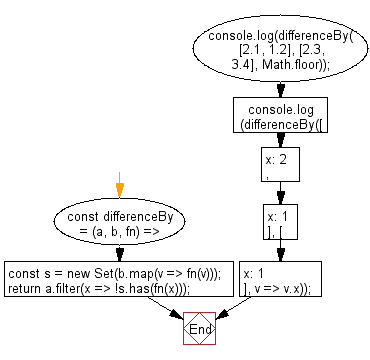# JavaScript: Return the difference between two arrays

## JavaScript fundamental (ES6 Syntax): Exercise-73 with Solution

Write a JavaScript program to return the difference between two arrays, after applying the provided function to each array element of both.

• Create a Set by applying fn to each element in b.
• Use Array.prototype.map() to apply fn to each element in a.
• Use Array.prototype.filter() in combination with fn on a to only keep values not contained in b, using Set.prototype.has().

Sample Solution:

JavaScript Code:

``````//#Source https://bit.ly/2neWfJ2
const differenceBy = (a, b, fn) => {
const s = new Set(b.map(v => fn(v)));
return a.filter(x => !s.has(fn(x)));
};
console.log(differenceBy([2.1, 1.2], [2.3, 3.4], Math.floor));
console.log(differenceBy([{ x: 2 }, { x: 1 }], [{ x: 1 }], v => v.x));
```
```

Sample Output:

```[1.2]
[{"x":2}]
```

Flowchart:Live Demo:

See the Pen javascript-fundamental-exercise-73 by w3resource (@w3resource) on CodePen.

Improve this sample solution and post your code through Disqus

What is the difficulty level of this exercise?

Test your Programming skills with w3resource's quiz.

﻿

## JavaScript: Tips of the Day

Returns the difference (in days) between two dates

Example:

```const tips_DiffBetweenDates = (dateInitial, dateFinal) =>
(dateFinal - dateInitial) / (1000 * 3600 * 24);
console.log(tips_DiffBetweenDates(new Date('2020-05-13'), new Date('2020-06-05')));
```

Output:

```23
```